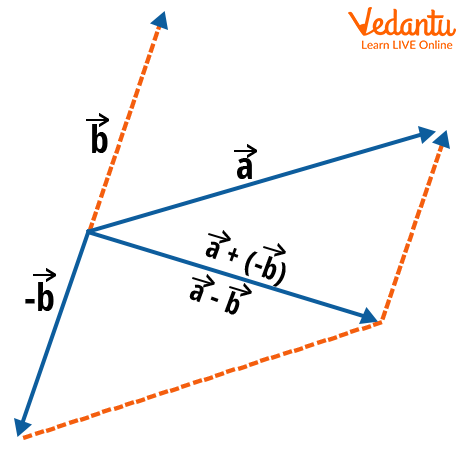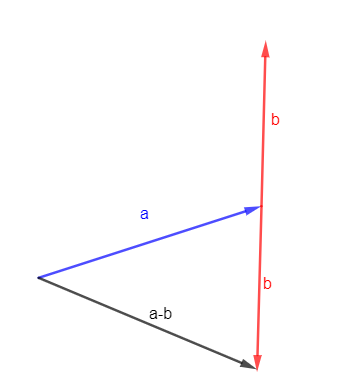Courses
Courses for Kids
Free study material
Free LIVE classes
More

# Subtraction of Vectors for JEE## What are Vectors?

Last updated date: 21st Mar 2023
Total views: 81.9k
Views today: 1.59k

A vector can be defined as a quantity, measurement, or object that has both a magnitude and a direction. It is one of the most important and basic concepts in Physics that finds its applications in almost all the branches of the subject. A vector can be visualised geometrically as a directed line segment. The length of the line segment corresponds to the magnitude of the vector and the arrowhead corresponds to the direction of the vector. The direction of any vector is defined from its tail to its head where the arrow is there.

Most common examples of vector quantities include force, velocity, acceleration, displacement, momentum, electric field, etc. Basic algebraic operations can be applied to vectors, but they have their own rules for these operations. We cannot add or subtract vectors like we add or subtract numbers. Vectors have directions too, so this has to be taken into consideration. We will look at how to subtract vectors in detail in this article.Image: Geometrical representation of a Vector

## What is Subtraction of Vectors?

Suppose there are vectors a and b. The subtraction of these vectors is represented as, a-b. In simple words, it is just the addition of the vector -b to the vector a. It can be written as,

a-b=a+(-b)

One can visualise vector subtraction as the addition of a vector to another vector after rotating it 180° in space. Subtraction of vectors involves the addition of vectors and the negative of any vector. It is obvious that the subtraction of two vectors will give a vector as the result. Two rules can be laid out for vector subtraction.

• Vector subtraction can be performed only between two vectors.

• The vectors which are being subtracted should represent the same physical quantity, otherwise, they cannot be subtracted.

## Parallelogram Law of Vector Subtraction

Let’s continue with the same vectors a and b. These vectors can be visualised in space as given in the diagram given below. To apply the parallelogram law the vectors have to be coterminal or their initial points should coincide.Image: Parallelogram Law of Vector Subtraction

The basic question before starting subtraction is how to understand what the subtraction of these vectors means. The subtraction is a-b and when b is added to this subtraction, the answer should be a. This can be shown as,

(a-b)+b=a

The above figure shows vectors a and b. Now subtraction basically means adding -b to a. If we rotate the vector b by 180°  and then add it to a, we’ll have our answer. The figure below helps visualise this process.

Now forming a parallelogram by forming lines around the two vectors will give a parallelogram as shown in the above figure. Then a diagonal drawn from the initial point of the vectors to the opposite vertex will give the resultant, in this case, the subtraction. So we have the resultant, a-b by using the parallelogram law.

## Triangle Law of Vector Subtraction

To use the triangle law of vector subtraction, the vectors don’t need to have the same initial point. The initial point of one vector needs to be at the terminal point of the previous vector. Then the resultant of the vectors, or the addition of those vectors will be drawn from the initial point of the first vector to the terminal point of the last vector. For subtraction, the second vector should be rotated by 180°  and then the triangle law can be applied to get the resultant or the subtraction of those vectors.

It can be visualised by using the figure below.Image: Triangle Law for Vector Subtraction

Here the vector b was rotated by 180° and then the resultant was drawn from the initial point of a to the tip of -b to get a-b.

## Vector Subtraction Formula

Let’s continue with vectors a and b. Apart from the graphical ways of subtracting the vectors, they can also be subtracted by subtracting their respective components from each other. Let,

a={a1, a2

b={b1, b2}

The respective components can be subtracted to get a-b.

a-b=a+(-b)

a-b={(a1-b1), (a2-b2)}

This is the vector subtraction formula. This can be extended to any number of components that the vector has.

Let’s take a vector subtraction example to understand things better.

Suppose a={3,5}, and b={2,6}.

The subtraction will be given as,

a-b={(3-2), (5-6)}

a-b={1, -1}

It is important to know that the subtraction of vectors obeys which laws. Subtraction of vectors obeys the distributive law, that is

a(b-c)=ab-ac

Here a, b, and c  are vectors.

### Conclusion

The subtraction of vectors is the same as adding the reverse or inverse of one vector to another vector. The parallelogram law of subtraction can be used to calculate the subtraction of the vectors by drawing a parallelogram taking the two vectors with the same initial point. Then the resultant can be drawn as a diagonal from the initial point to the opposite vertex.

Similarly, the triangle law can also be used to find the subtraction of vectors. The vectors have to be arranged by keeping the initial point of the second vector on the tip of the first vector and then drawing the resultant from the initial point of the first vector to the tip of the second vector. Also, the individual components can be subtracted to get the subtraction.

## FAQs on Subtraction of Vectors for JEE

1. How do you add and subtract multiple vectors?

Multiple vectors can be subtracted in the same way used to subtract two vectors. Taking two vectors at a time and then repeating this process will allow subtracting any number of vectors. The parallelogram law of subtraction can also be used to subtract multiple vectors by arranging them in the form of a parallelogram and then forming diagonals from the initial points and then using this diagonal as one vector and subtracting another vector from it.

2. Is commutative law applicable to vector subtraction?

No, the commutative law does not apply to vector subtraction. Commutative law for the subtraction will mean that, a-b=b-a. This is applicable for the subtraction of numbers or scalars in general, but this law cannot be applied to vector subtraction because when a-b is calculated, b  is rotated by  180° and when b-a is calculated, a  is rotated by 180° which are not equal to each other. So, the commutative law is not applicable for vector subtraction.